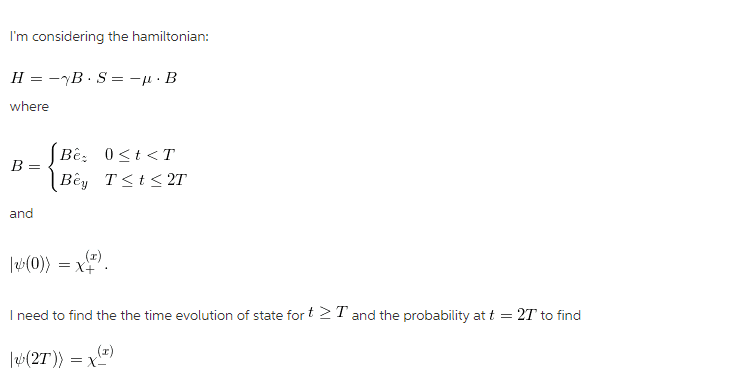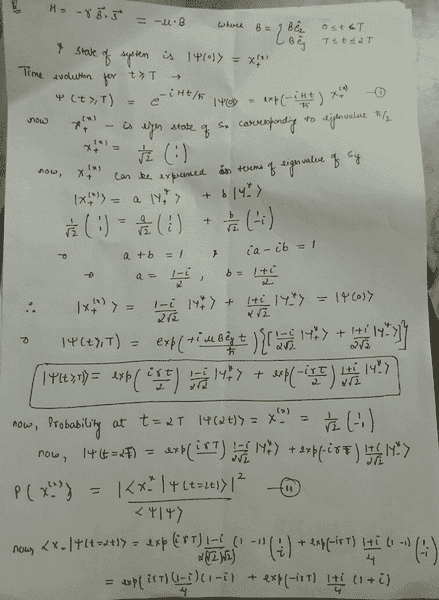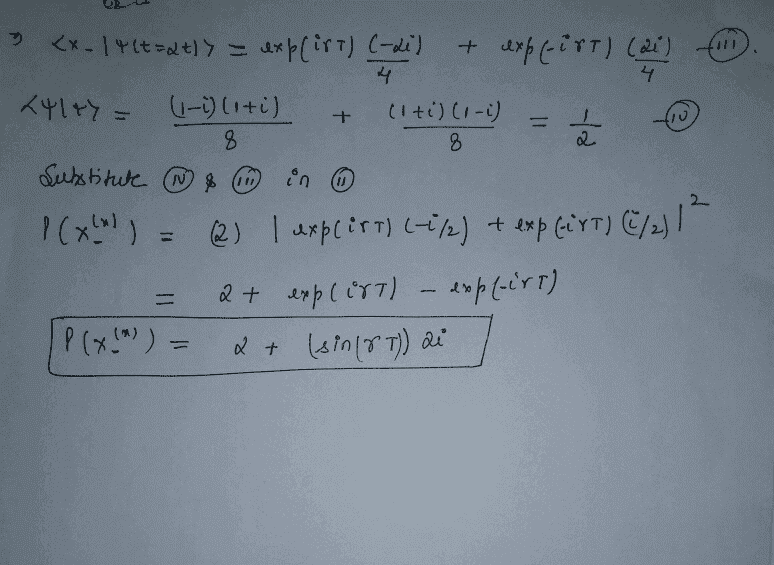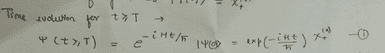# Quantum version of Larmor precession

• Bobs
In summary, there is a conversation about a problem involving a quantum system evolving under different magnetic fields. The initial state of the system is given and the problem involves finding the probability of the system being in a certain state at a certain time. There is some confusion about the correct way to approach the problem and different answers are being proposed. The correct probability is still being determined. f

## Homework Statement## Homework Equations

I didn't get what this actually means.

## The Attempt at a SolutionI'm not sure whether it is correct. Could you take a look?
Regards!

#### Attachments

Hello. I haven't gone through all of the details of your calculations. But, I don't see where you have taken into account the evolution of the system between ##t = 0## and ##t = T##. It appears to me that you are assuming that the system is in state ##\chi_+^{(x)}## at time ##t = T##.#### Attachments

Do you see any mistakes at the final answer? And yes, I'm assuming that the system in state ##\chi_+^{(x)}## at time ##t=T##. Staying tuned for your sincerely reply!

Maybe we are interpreting the statement of the problem differently. The way I read it, at time ##t = 0## the system is in state ##|\psi(0) \rangle = \chi_+^{(x)}##. It evolves for time ##T## with ##\mathbf B## in the z direction. Then, in the time interval ##T \leq t \leq 2T## it evolves with ##\mathbf B## in the y direction. So, you will need to find ##|\psi(T) \rangle## before you can determine the state for ##t > T##.

Your final result for the probability appears to be a complex number. But probabilities are real numbers. Also, shouldn't the answer depend on the magnetic field strength B?

It looks like this post has appeared twice.
Do you see any mistakes at the final answer? And yes, I'm assuming that the system in state at time ##t=T##. Staying tuned for your sincerely reply!
The systaem will not be in state ##\chi_+^{(x)}## at t = T. As TSny points out in the above post, the evolution between t = 0 and t = T is dictated by the magnetic field only in the z direction. You should calculate |ψ(T)> from that, and then let that evolve from t = T to t = 2T with the magnetic field only in the y direction.

I found the probability as ##\dfrac{B^2}{2}## is that correct?

I found the probability as ##\dfrac{B^2}{2}## is that correct?
That answer can't possibly be correct. Probabilities are unitless.

Finally, I've found the probability as ##0## from ##P = \dfrac{1}{4}exp \biggr( \dfrac{-2t\mu BB}{\hbar}\biggr ) \biggr | (1-1) \biggr |^2 = 0## Does that seem correct now? Thanks in advance.